# SAT Math Multiple Choice Question 899: Answer and Explanation

### Test Information

Question: 899

14. Given that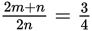, which of the following must also be true?

• A.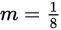• B.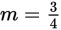• C.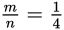• D.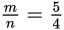Explanation:

C

Difficulty: Medium

Category: Passport to Advanced Math / Exponents

Strategic Advice: Whenever you see only one equation, but two variables, chances are that you can't solve for either variable. Rather, you're most likely looking for an answer that somehow compares the two variables. Notice that the first two answer choices give a solution for m, which is probably not possible to find. The last two choices give a ratio of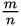, so try to write the original equation with at least onein it.

Getting to the Answer: Start by breaking the fraction on the left side of the equation into two parts. Then, simplify and see what's left: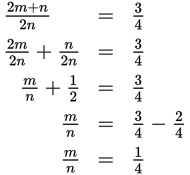This matches (C).